#Function Repository Resource:

# LuckyNumbers

Get a list of lucky numbers

Contributed by: Enrique Zeleny
 ResourceFunction["LuckyNumbers"][n] gives the lucky numbers until integer n. ResourceFunction["LuckyNumbers"][n,k] gives the lucky numbers until integer n, only iterating the sieve procedure k times.

## Details and Options

Lucky numbers are defined through a sieving procedure: Start with the odd integers and strike out every third number. This gets rid of 5, 11, 17, 23 and so on. The third surviving number from the left is 7, so strike out every seventh integer not yet eliminated: 19, 39, .... The integers that survive are the lucky numbers.
Lucky numbers seem to share many properties with primes.

## Examples

### Basic Examples

First lucky numbers before 100:

 In:=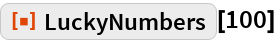Out=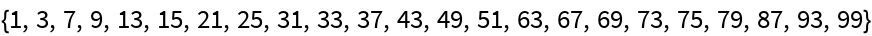Lucky numbers that are primes are lucky primes:

 In:=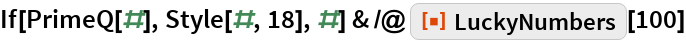Out=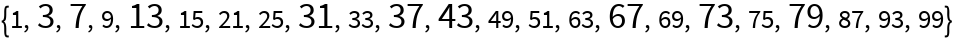The first 300 integers surviving 6 steps of the lucky sieve process:

 In:=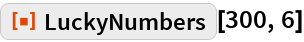Out=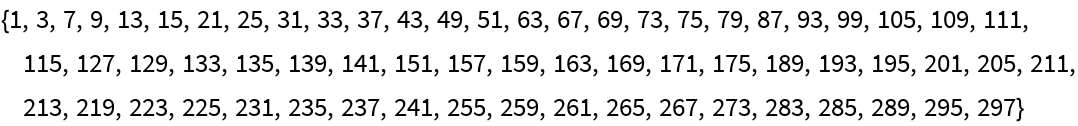Step-by-step sieve for lucky numbers:

 In:=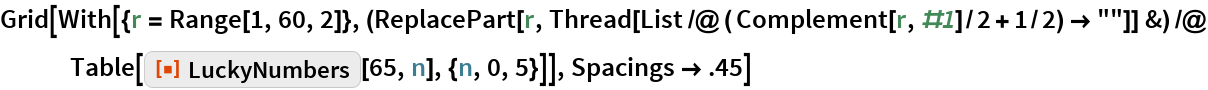Out=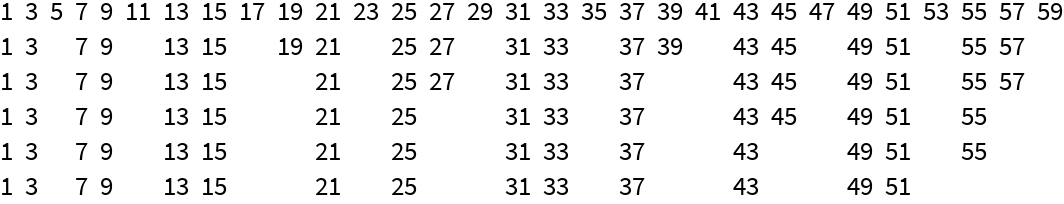In:=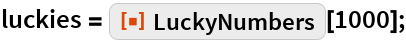Distance between lucky numbers:

 In:=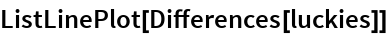Out=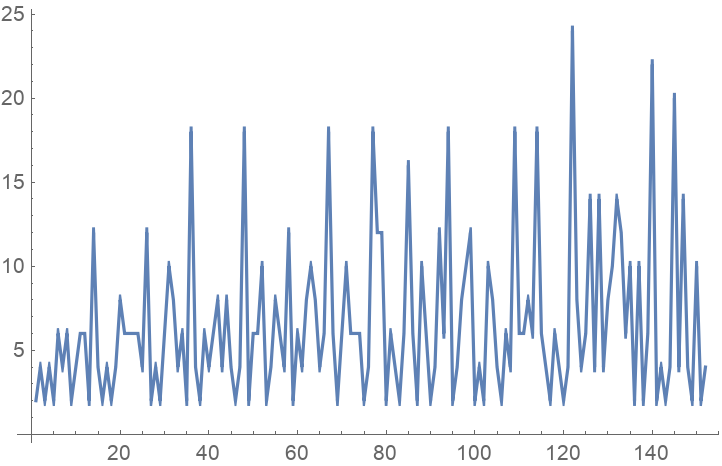Highlight lucky numbers in an array:

 In:=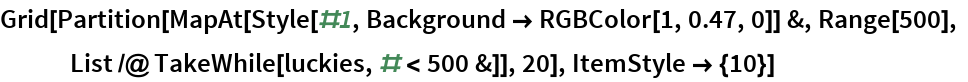Out=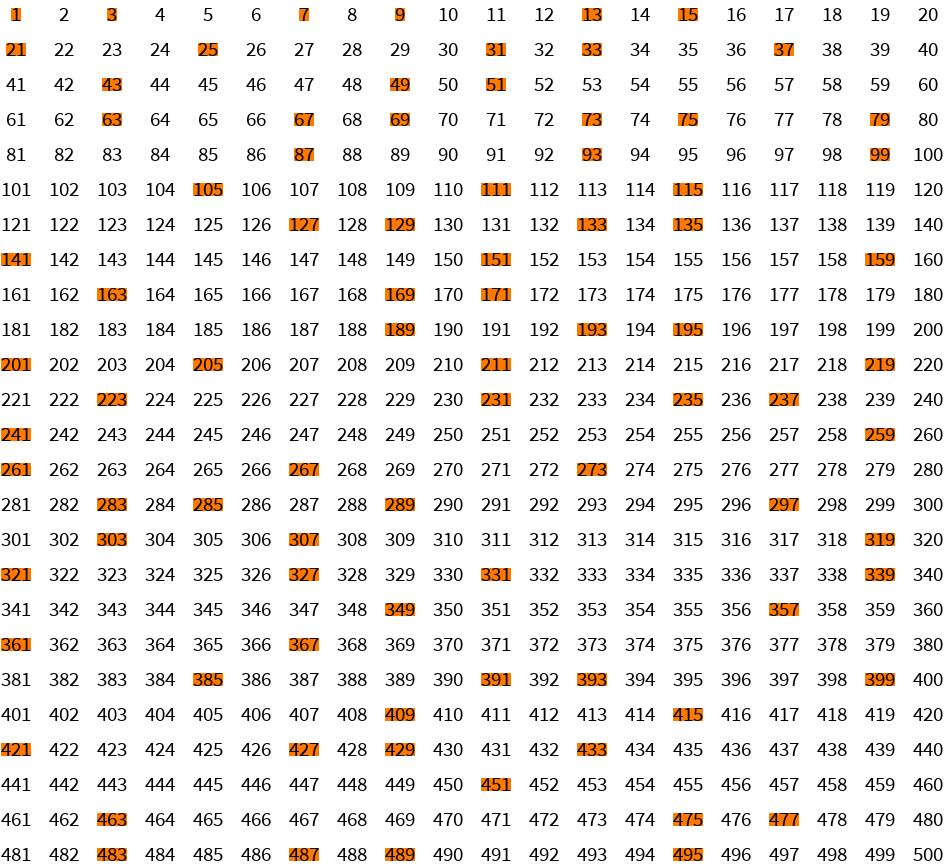Comparing with prime numbers:

 In:=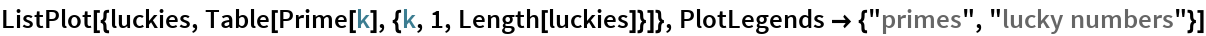Out=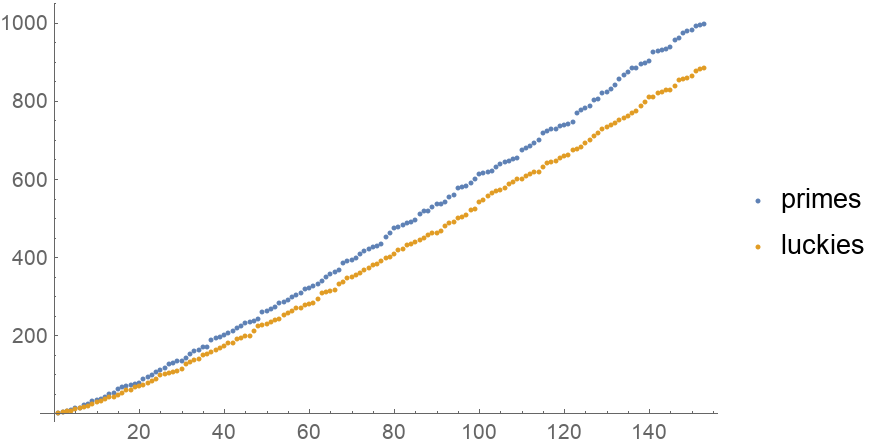“Twin” lucky numbers (those separated by an even number):

 In:=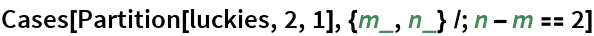Out=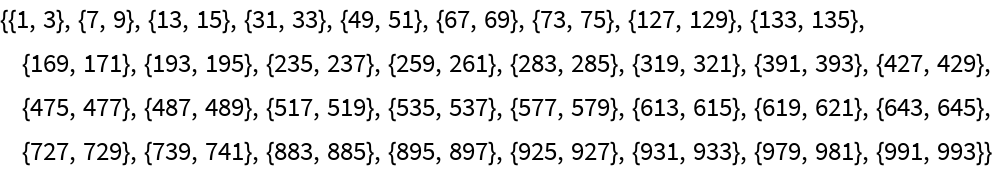Goldbach conjecture for luckies (an even number is a sum of two luckies):

 In:=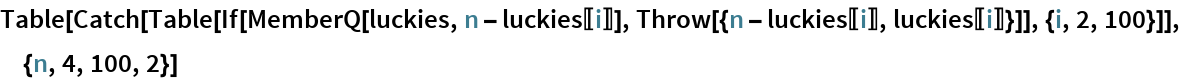Out=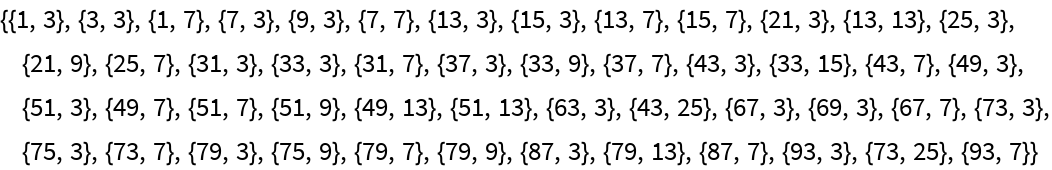Number of lucky numbers less than a million:

 In:=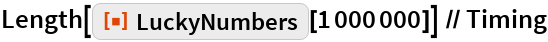Out=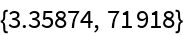## Requirements

Wolfram Language 11.3 (March 2018) or above